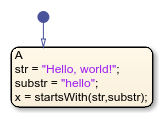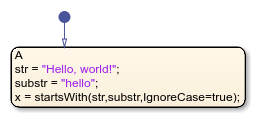# startsWith

## Syntax

``tf = startsWith(str,pat)``
``tf = startsWith(str,pat,IgnoreCase=true)``

## Description

example

````tf = startsWith(str,pat)` returns `1` (`true`) if `str` starts with the specified pattern, and returns `0` (`false`) otherwise.```

example

````tf = startsWith(str,pat,IgnoreCase=true)` ignores case when determining if `str` starts with `pat`. ```

Note

Only Stateflow® charts that use MATLAB® as the action language support the `startsWith` operator.

## Examples

expand all

```h = "Hello, world!" pat1 = "H" pat2 = "h" x = startsWith(h,pat1) x2 = startsWith(h,pat2) ``````h = "Hello, world!" pat1 = "H" pat2 = "h" x = startsWith(h,pat1,IgnoreCase=true) x2 = startsWith(h,pat2,IgnoreCase=true) ```## Version History

Introduced in R2021b